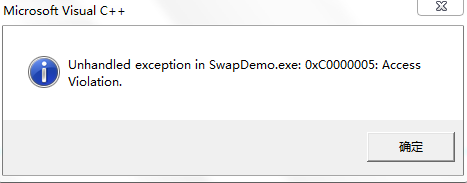# 重拾C++经典笔试30题（11-20）

11. C++对象模型基本概念之程序使用内存区

 数据区存储分类 存储内容 全局/静态数据区 全局变量及静态变量（全局静态变量、局部静态变量） 常量数据区 存储程序中的常量字符串等 栈 存储自动变量或局部变量，以及传递的函数参数等 堆 用户控制的存储区，存储动态产生的数据 代码区 程序中代码

12.不被重视的sizeof()大小问题。

(1)情况一

int a = 8;

cout<< sizeof(a=6) <<endl; //a=6是不被编译的，只是转换为a的类型。

cout<< a<< endl; //8

(2)情况二，求函数大小等价于其对应返回值的大小。

int fun1()
{
return 0;
}

void fun2()
{

}

char fun3()
{
return 'c';
}

double fun4()
{
return 0.0;
}

cout << sizeof(fun1()) <<endl; //4
cout << sizeof(fun2()) <<endl; //error C2070:illegal sizeof operand**
cout << sizeof(fun3()) <<endl; //1
cout<< sizeof(fun4()) <<endl; //8



(3)情况三，求数组大小。

char c ={"a",""};

cout <<sizeof(c) << endl; //2*3*1

(4)情况四，括号、给数组大小赋值（主要原因，编译的时候已经计算过sizeof大小了）。

        int na = 35;
//等价于sizeof(na),如果是变量名，可以不加括号。
cout << sizeof na << endl;
cout << sizeof(int)<<endl;

int nArray = {0};
//可以通过sizeof( )定义数组的大小，等价于new int.
int *pArray = new int[sizeof(nArray)];



13.深究结构体地址对齐的原则及应用实例

struct simpleA
{
float f; //0
char p; //4
};//8+12-->20



(1)每个成员分别按自己的方式对齐,并能最小化长度

(2).复杂类型(如结构)的默认对齐方式是它最长的成员的对齐方式,这样在成员是复杂类型时，可以最小化长度；

(3).对齐后的长度必须是成员中最大的对齐参数的整数倍，这样在处理数组时可以保证每一项都边界对齐

14.注意此处错误的原因

void swap1(int* p ,int* q)
{
int* temp; //单纯的temp会出现temp' used withouthaving beeninitialized
*temp = *p;
*p = *q;
*q = *temp;
}15.地址相减计算

int main()
{
int a;
a = 0;
a = 1;
a = 2;

int *p, *q;
p = a;
q = &a;
cout << p << endl;
cout << q << endl;

//q-p等价于[(q的地址值-p的地址值)/sizeof(int)].
cout << q-p << endl; //2
cout << a[q-p] <<endl;//a = 2;

return 0;
}



16.为什么是1

class A
{
public:
A() { m_a = 1, m_b =2; }
~A(){}
void fun() { printf("%d \t%d\n", m_a, m_b); }
int m_a;
int m_b;
};
class B
{
public:
B() { m_c = 3;}
~B(){}
void fun() {printf("%d\n",m_c); } //为什么是1，思考！
int m_c;
};
int main()
{
A a;
B *pb = (B*)&a;
pb->fun(); //为什么是1，思考！
cout << &a<<endl; //12FF6C
cout << &(a.m_a)<<endl; //12FF6C
printf("%08x\n",&A::m_a);
printf("%08x\n",&A::m_b);
printf("%08x\n",&B::m_c);
return 0;
}



17.一个含有10个指针的数组，该指针指向一个函数，该函数有一个整形参数并返回一个整形数。

int(*p)(int)

18.有了malloc/free为什么还用new/delete?

1)malloc/freeC/C++标准库函数；new/deleteC++运算符。他们都可以申请和释放动态内存。

2)只用malloc/free无法满足非内部数据类型的要求；对象在创建的时候自动调用构造函数，在销毁的时候自动调用析构函数；而malloc/free是库函数而不是运算符，不再编译器控制权限之内，所以不能把调用构造函数和析构函数的任务强加给它们。

19.注意下列的取值

int main()
{
int a[] = {1,2,3,4,5};
int *ptr = (int*)(&a+1); //1代表1个sizeof(a)
printf("%d%d\n",*(a+1),*(ptr-1));//2 , 5
return 0;
}

int main()
{
char* a[]={"hello","the","word"};
char** pa = a; //pa为指向字符串数组的指针.
pa++;
cout << *pa << endl; //the
cout << *pa << endl;//t
cout << *(*pa+1)<<endl;//h
cout << *(*pa+2) <<endl;//e

return 0;

}



20.题解：深拷贝+浅显拷贝，为什么？

class CA
{

public:

CA(int b,char* cstr)
{

cout <<"CAconstructor!" << endl;
a=b;
str=new char[b];
strcpy(str,cstr);
}

void Show()
{
cout<<str<<endl;
}

~CA()
{
cout <<"~CAconstructor!" << endl;
delete str;
}

private:
int a;
char *str;

};

int main()
{

CA A(10,"Hello!");
CA B=A; //此处会调用默认的拷贝构造函数，是为浅拷贝。
B.Show();

//浅拷贝后A，B对象的str字符串是同一个地址。当发生析构时会出现运行报错！

return 0;
}



//对比浅拷贝，以下是深拷贝。

#include"stdafx.h"
#include<iostream>
usingnamespace std;

class CA
{
public:
CA(int b,char* cstr)
{
cout <<"CAconstructor!" << endl;
a=b;
str=new char[b];
strcpy(str,cstr);
}

//自定义拷贝构造函数

CA(constCA& C)
{
a=C.a;
str=newchar[a]; //深拷贝
if(str!=0)
strcpy(str,C.str);
}

void Show()
{
cout<<str<<endl;
}

~CA()
{

cout <<"~CAconstructor!" << endl;
delete str;
}

private:
int a;
char *str;
};

int main()
{

CA A(10,"Hello!");
CA B=A;
B.Show();
return 0;
}



09-02333412-034623
08-091万+
10-142930
09-0944
04-191222
11-30
10-207628
©️2020 CSDN 皮肤主题: Age of Ai 设计师:meimeiellie